## Confidence Interval

The probability that a measurement will fall within a given Closed Interval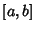. For a continuous distribution,(1)

whereis the Probability Distribution Function. Usually, the confidence interval of interest is symmetrically placed around the mean, so(2)

where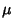is the Mean. For a Gaussian Distribution, the probability that a measurement falls within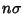of the meanis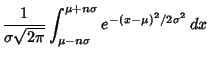(3)

Now let, so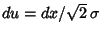. Then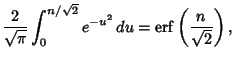(4)

whereis the so-called Erf function. The variate value producing a confidence interval CI is often denoted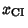, so(5)

 range CI0.68268950.95449970.9973002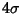0.99993660.9999994

To find the standard deviation range corresponding to a given confidence interval, solve (4) for.(6)

 CI range 0.800 ± 1.281550.900 ± 1.644850.950 ± 1.959960.990 ± 2.575830.995 ± 2.807030.999 ± 3.29053